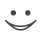# This flashcard is just one of a free flashcard set. See all flashcards!

219
When moving from valuing an option on a non-dividend paying stock to an option on a currency which of the following is true?
A.The risk-free rate is replaced by the excess of the domestic risk-free rate over the foreign risk-free rate in all calculations
B.The formula for u changes
C.The risk-free rate is replaced by the excess of the domestic risk-free rate over the foreign risk-free rate for discounting
D.The risk-free rate is replaced by the excess of the domestic risk-free rate over the foreign risk-free rate when p is calculated